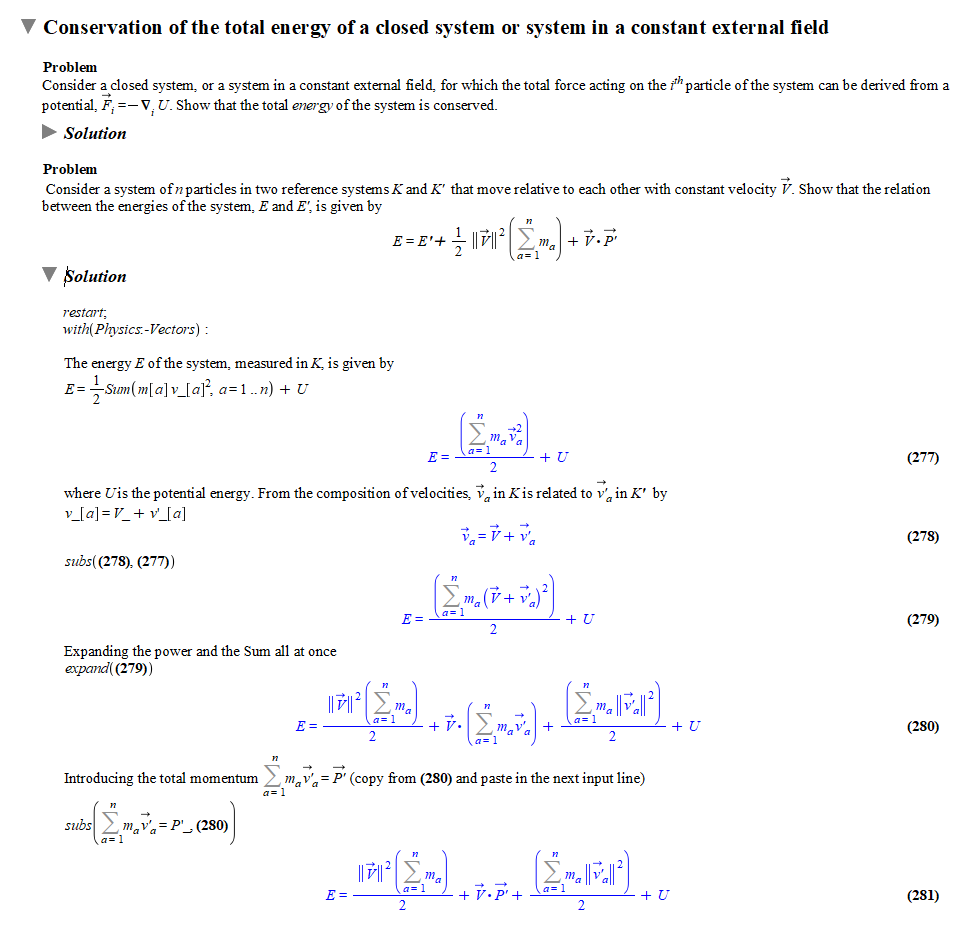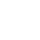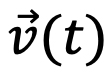Math Software for Classical Mechanics

# Math Software for Classical Mechanics

Includes a comprehensive collection of examples covering standard introductory courses and beyond

## Finally, math software that works for physics, too!

While math, engineering, and many science courses can easily leverage math software to ease the computational burden that can interfere with conceptual learning, the same hasn't always been true for physics. Tools designed for math haven't worked nearly as well in physics courses due to the subject's heavy use of notation and differing conventions.

But times have changed! In recent years, the math software Maple has added comprehensive support for the notation, conventions, and computations used by physicists, providing a natural environment for computer-assisted physics computations. Now you and your students can focus on concepts and problem-solving techniques, while leaving the time-consuming, error-prone algebraic computations and manipulations to Maple. With Maple, you can:

• Keep students focused on the physics, not the algebraic manipulations
• Work through more examples and more complex problems in class, without using up too much class time
• Explore what-if scenarios on-the-fly by modifying problems and easily recomputing the solutions
• Give students more opportunities to practice by giving them a tool that lets them try extra problems and check answers on their own
• Build student confidence by eliminating the mechanical mistakes that can lead to students incorrectly feeling that they have not understood the fundamentals when all they've really done is a lost a minus sign

## Comprehensive Content for Mechanics Courses

To make it easy for you and your students to obtain these benefits quickly, Maple now includes an extensive collection of content to support introductory courses in Classical Mechanics, and beyond.

• Over 65 fully worked problems and solutions demonstrate how to use Maple throughout the course
• Comprehensive topic list includes working with curvilinear coordinates, equations of motion (vector and tensor notation), conservation laws, motion in a central field, small oscillations, rigid body motion, inertia tensor and non-inertial reference frames.
See full list of topics
• Examples are easy to modify so you can use them to explore what-if scenarios and as a starting point for solving similar problems
• Uses standard physics notation, so problems can be entered easily and results are easy to interpret
• Compatible with any standard textbook## See it for yourself, right now!

Explore the Mechanics content in 3 easy steps:

2. In Maple, enter “Mechanics” in the Maple search box and select the first entry (Physics,Courseware….) to open the content as a help page
3. To run or modify the examples, click on thebutton in the toolbar to open the help page as a worksheet

## List of Topics

1. Position, velocity and acceleration in Cartesian, cylindrical and spherical coordinates
• The positionas a function of time
• The velocity• The acceleration• Deriving these formulas
• Velocity and acceleration in the case of 2-dimensional motion on the x, y plane
2. The equations of motion
• A single particle
• The equations of motion - vectorial form
• The case of constant accelerationo The case of constant acceleration
• Motion under gravitational force close to the Earth's surface
• Motion under gravitational force not close to the Earth's surface
• Circular motion
• Escape velocity
• Different acceleration in different regions
• The equations of motion using tensor notation
• Cartesian coordinates
• Curvilinear coordinates
• Many-particle systems
• Center of mass
• The equations of motion
• Static: reactions of planes and tensions on cables
• Lagrange equations
• Motion of a pendulum
3. Conservation laws
• Work
• Conservation of the total energy of a closed system or a system in a constant external field
• Conservation of the total momentum of a closed system
• Conservation of angular momentum
• Cyclic coordinates
4. Integration of the equations of motion
• Motion in one dimension
• Reduced mass
• The two-body problem
• A many-body problem
• Motion in a central field
• Kepler's problem
5. Small Oscillations
• Free oscillations in one dimension
• Forced oscillations
• Oscillations of systems with many degrees of freedom
6. Rigid-body motion
• Angular velocity
• Inertia tensor
• Angular momentum of a rigid body
• The equations of motion of a rigid body
7. Non-inertial coordinate systems
• Coriolis force and centripetal force